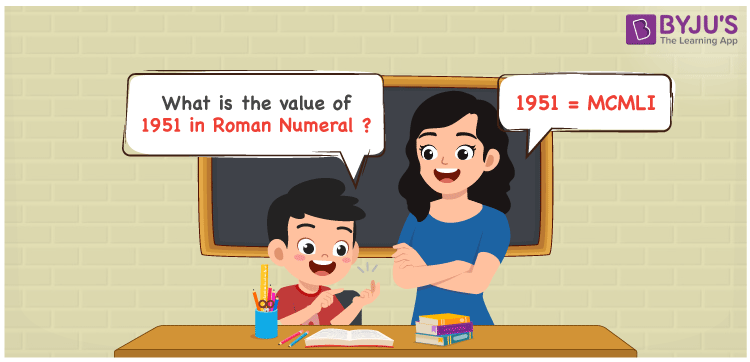# 1951 in Roman Numerals

1951 in Roman Numerals is MCMLI. Roman numerals is an additive and subtractive system where letters are used to show certain numbers in the number system. A different combination of symbols are used to indicate the roman numerals that include the English alphabet. Therefore, the 1951 can be written as MCMLI in roman numerals.

 Number Roman Numeral 1951 MCMLI## How to Write 1951 in Roman Numerals?

In order to write 1951 in Roman Numerals, it has to be written in expanded form, i.e.

1951 = 1000 + (1000 – 100) + 50 + 1

1951 = M + (M – C) + L + I

1951 = MCMLI

## Video Lesson on Roman Numerals## Frequently Asked Questions on 1951 in Roman Numerals

Q1

### Write 1951 in Roman Numerals.

In Roman Numerals, 1951 is written as MCMLI.
Q2

### Write the numbers 1950 to 1959 in roman numerals.

MCML = 1000 + 900 + 50 = 1950

MCMLI = 1000 + 900 + 50 + 1 = 1951

MCMLII = 1000 + 900 + 50 + 2 = 1952

MCMLIII = 1000 + 900 + 50 + 3 = 1953

MCMLIV = 1000 + 900 + 50 + 4 = 1954

MCMLV = 1000 + 900 + 50 + 5 = 1955

MCMLVI = 1000 + 900 + 50 + 6 = 1956

MCMLVII = 1000 + 900 + 50 + 7 = 1957

MCMLVIII = 1000 + 900 + 50 + 8 = 1958

MCMLIX = 1000 + 900 + 50 + 9 = 1959

Q3

### What does 1951 in Roman Numerals Mean?

To write 1951 in roman numerals, we will first express 1951 in expanded form.

1951 = 1000 + (1000 – 100) + 50 + 1 = M + (M – C) + L + I = MCMLI. Hence, 1951 in roman numbers is expressed as MCMLI.